# Disease Outbreak Response Decision-making Under Uncertainty: A retrospective analysis of measles in Sao Paulo¶

In :
%matplotlib inline
import pandas as pd
import numpy as np
import numpy.ma as ma
from datetime import datetime
import matplotlib.pyplot as plt
import seaborn as sb
sb.set()
import pdb

from IPython.core.display import HTML
def css_styling():
styles = open("styles/custom.css", "r").read()
return HTML(styles)
css_styling()

/usr/local/lib/python3.4/dist-packages/matplotlib/__init__.py:872: UserWarning: axes.color_cycle is deprecated and replaced with axes.prop_cycle; please use the latter.
warnings.warn(self.msg_depr % (key, alt_key))

Out:
In :
data_dir = "data/"


Import outbreak data

In :
measles_data = pd.read_csv(data_dir+"measles.csv", index_col=0)
measles_data.BIRTH = pd.to_datetime(measles_data.BIRTH)
measles_data.ONSET = pd.to_datetime(measles_data.ONSET)

In :
measles_data = measles_data.replace({'DISTRICT': {'BRASILANDIA':'BRAZILANDIA'}})


Sao Paulo population by district

In :
sp_pop = pd.read_csv(data_dir+'sp_pop.csv', index_col=0)

In :
_names = sp_pop.index.values
_names[_names=='BRASILANDIA'] = 'BRAZILANDIA'
sp_pop.set_index(_names, inplace = True)

In :
sp_pop.head()

Out:
0 a 4 anos 5 a 9 anos 10 a 14 anos 15 a 19 anos 20 a 24 anos 25 a 29 anos 30 a 34 anos 35 a 39 anos 40 a 44 anos 45 a 49 anos 50 a 54 anos 55 a 59 anos 60 a 64 anos 65 a 69 anos 70 a 74 anos 75 anos e + Total
AGUA RASA 5411 5750 6450 7122 7621 7340 6999 6984 6346 5608 4987 4212 4152 3595 2937 3637 89151
ALTO DE PINHEIROS 2070 2369 2953 3661 4612 4190 3539 3633 3448 3289 3040 2533 2298 1732 1305 1823 46495
ANHANGUERA 3068 3006 2755 2431 2426 2636 2695 2308 1653 1107 753 509 352 217 162 171 26249
ARICANDUVA 7732 7730 8373 8956 9182 8531 7813 7365 6551 5554 4887 3858 3320 2449 1611 1723 95635
ARTUR ALVIM 9031 9078 10000 11058 11387 10347 9125 8658 7830 7055 5919 4612 3756 2633 1727 1724 113940

Plot of cumulative cases by district

In :
measles_onset_dist = measles_data.groupby(['DISTRICT','ONSET']).size().unstack(level=0).fillna(0)
measles_onset_dist.cumsum().plot(legend=False, grid=False)

Out:
<matplotlib.axes._subplots.AxesSubplot at 0x7f465cc31358>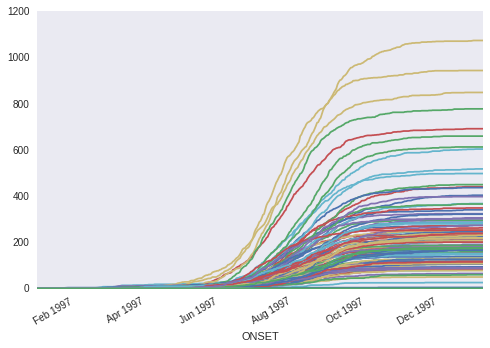In :
total_district_cases = measles_onset_dist.sum()


Top 5 districts by number of cases

In :
totals = measles_onset_dist.sum()
totals.sort(ascending=False)
totals[:5]

/usr/local/lib/python3.4/dist-packages/ipykernel/__main__.py:2: FutureWarning: sort is deprecated, use sort_values(inplace=True) for for INPLACE sorting
from ipykernel import kernelapp as app

Out:
DISTRICT
GRAJAU             1074
JARDIM ANGELA       944
CAPAO REDONDO       849
JARDIM SAO LUIZ     778
CAMPO LIMPO         692
dtype: float64

Age distribution of cases, by confirmation status

In :
by_conclusion = measles_data.groupby(["YEAR_AGE", "CONCLUSION"])
counts_by_cause = by_conclusion.size().unstack().fillna(0)
ax = counts_by_cause.plot(kind='bar', stacked=True, xlim=(0,50), figsize=(15,5))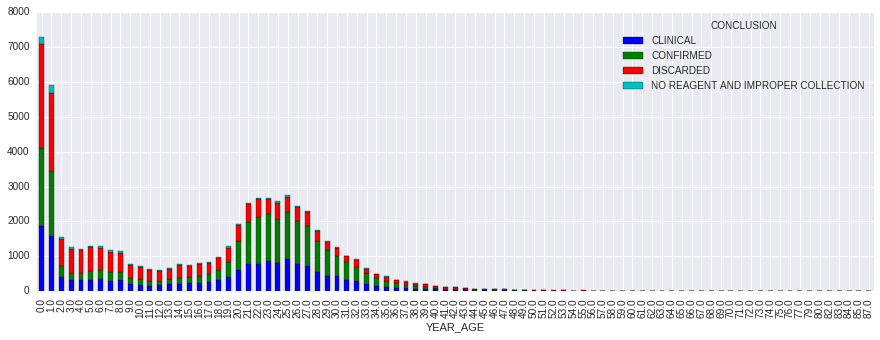### Vaccination Data¶

In :
vaccination_data = pd.read_csv('data/BrazilVaxRecords.csv', index_col=0)

Out:
BIRTHS VAX POP SIA
YEAR
1980 3896442 0.57 121740438 0
1981 3933136 0.73 124610790 0
1982 3952137 0.66 127525420 0
1983 3952735 0.68 130455659 0
1984 3935224 0.73 133364277 0
In :
vax_97 = np.r_[*(1979-1921+1), vaccination_data.VAX[:17]]
n = len(vax_97)
FOI_mat = np.resize((1 - vax_97*0.9), (n,n)).T

In :
vacc_susc = (1 - vax_97*0.9)[::-1]
vacc_susc = 0.5

In :
sia_susc = np.ones(len(vax_97))
birth_year = np.arange(1922, 1998)[::-1]
by_mask = (birth_year > 1983) & (birth_year < 1992)


## Stochastic Disease Transmission Model¶

As a baseline for comparison, we can fit a model to all the clinically-confirmed cases, regardless of lab confirmation status. For this, we will use a simple SIR disease model, which will be fit using MCMC.

This model fits the series of 2-week infection totals in each district $i$ as a set of Poisson models:

$$Pr(I(t)_{i} | \lambda(t)_i) = \text{Poisson}(\lambda(t)_i)$$

Where the outbreak intensity is modeled as:

$$\lambda(t)_i = \beta [I^{(w)}(t-1)_i]^{\alpha} S(t-1)_i$$

$$\alpha \sim \text{Exp}(1)$$

We will assume here that the transmission rate is constant over time (and across districts):

$$\beta \sim \text{Gamma}(1, 0.1)$$

To account for the influence of infected individuals from neighboring districts on new infections, the outbreak intensity was modeled using a spatial-weighted average of infecteds across districts, where populations were weighted as an exponential function of the distance between district centroids:

$$w_{d} = \text{exp}(-\theta d)$$

$$\theta \sim \text{Exp}(1)$$

### Confirmation Sub-model¶

Rather than assume all clinical cases are true cases, we can adjust the model to account for lab confirmation probability. This is done by including a sub-model that estimates age group-specific probabilities of confirmation, and using these probabilities to estimate the number of lab-confirmed cases. These estimates are then plugged into the model in place of the clinically-confirmed cases.

We specified a structured confirmation model to retrospectively determine the age group-specific probabilities of lab confirmation for measles, conditional on clinical diagnosis. Individual lab confirmation events $c_i$ were modeled as Bernoulli random variables, with the probability of confirmation being allowed to vary by age group:

$$c_i \sim \text{Bernoulli}(p_{a(i)})$$

where $a(i)$ denotes the appropriate age group for the individual indexed by i. There were 16 age groups, the first 15 of which were 5-year age intervals $[0,5), [5, 10), \ldots , [70, 75)$, with the 16th interval including all individuals 75 years and older.

Since the age interval choices were arbitrary, and the confirmation probabilities of adjacent groups likely correlated, we modeled the correlation structure directly, using a multivariate logit-normal model. Specifically, we allowed first-order autocorrelation among the age groups, whereby the variance-covariance matrix retained a tridiagonal structure.

\begin{aligned} \Sigma = \left[{ \begin{array}{c} {\sigma^2} & {\sigma^2 \rho} & 0& \ldots & {0} & {0} \\ {\sigma^2 \rho} & {\sigma^2} & \sigma^2 \rho & \ldots & {0} & {0} \\ {0} & \sigma^2 \rho & {\sigma^2} & \ldots & {0} & {0} \\ \vdots & \vdots & \vdots & & \vdots & \vdots\\ {0} & {0} & 0 & \ldots & {\sigma^2} & \sigma^2 \rho \\ {0} & {0} & 0 & \ldots & \sigma^2 \rho & {\sigma^2} \end{array} }\right] \end{aligned}

From this, the confirmation probabilities were specified as multivariate normal on the inverse-logit scale.

$$\text{logit}(p_a) = \{a\} \sim N(\mu, \Sigma)$$

Priors for the confirmation sub-model were specified by:

\begin{aligned} \mu_i &\sim N(0, 100) \\ \sigma &\sim \text{HalfCauchy}(25) \\ \rho &\sim U(-1, 1) \end{aligned}

Age classes are defined in 5-year intervals.

In :
age_classes = [0,5,10,15,20,25,30,35,40,100]
measles_data.dropna(subset=['YEAR_AGE'], inplace=True)
measles_data['YEAR_AGE'] = measles_data.YEAR_AGE.astype(int)
measles_data['AGE_GROUP'] = pd.cut(measles_data.AGE, age_classes, right=False)


Lab-checked observations are extracted for use in estimating lab confirmation probability.

In :
CONFIRMED = measles_data.CONCLUSION == 'CONFIRMED'
CLINICAL = measles_data.CONCLUSION == 'CLINICAL'


Extract confirmed and clinical subset, with no missing county information.

In :
lab_subset = measles_data[(CONFIRMED | CLINICAL) & measles_data.COUNTY.notnull()].copy()

In :
age = lab_subset.YEAR_AGE.values
ages = lab_subset.YEAR_AGE.unique()
counties = lab_subset.COUNTY.unique()
y = (lab_subset.CONCLUSION=='CONFIRMED').values

In :
_lab_subset = lab_subset.replace({"CONCLUSION": {"CLINICAL": "UNCONFIRMED"}})
by_conclusion = _lab_subset.groupby(["YEAR_AGE", "CONCLUSION"])
counts_by_cause = by_conclusion.size().unstack().fillna(0)
ax = counts_by_cause.plot(kind='bar', stacked=True, xlim=(0,50), figsize=(15,5), grid=False)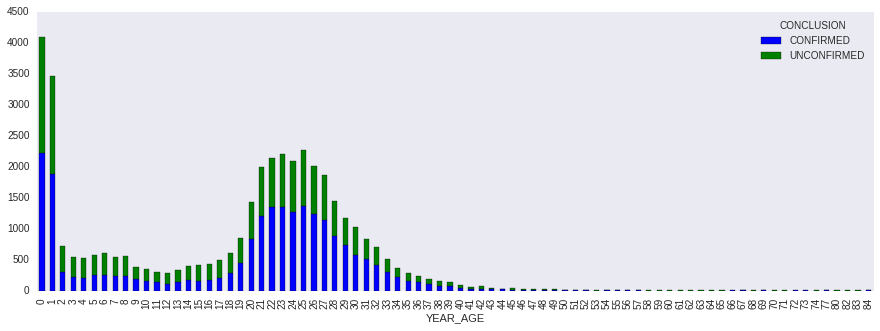In :
lab_subset.shape

Out:
(39982, 16)
In :
y.sum()

Out:
22097

Proportion of lab-confirmed cases older than 20 years

In :
(measles_data[CONFIRMED].YEAR_AGE>20).mean()

Out:
0.60257048468117846
In :
age_classes

Out:
[0, 5, 10, 15, 20, 25, 30, 35, 40, 100]
In :
age_group = pd.cut(age, age_classes, right=False)
age_index = np.array([age_group.categories.tolist().index(i) for i in age_group])
age_groups = age_group.categories
age_groups

Out:
Index(['[0, 5)', '[5, 10)', '[10, 15)', '[15, 20)', '[20, 25)', '[25, 30)',
'[30, 35)', '[35, 40)', '[40, 100)'],
dtype='object')
In :
#Extract cases by age and time.
age_group = pd.cut(age, age_classes, right=False)
age_index = np.array([age_group.categories.tolist().index(i) for i in age_group])

In :
age_group.categories

Out:
Index(['[0, 5)', '[5, 10)', '[10, 15)', '[15, 20)', '[20, 25)', '[25, 30)',
'[30, 35)', '[35, 40)', '[40, 100)'],
dtype='object')
In :
age_slice_endpoints = [g[1:-1].split(',') for g in age_groups]
age_slices = [slice(int(i), int(i)) for i in age_slice_endpoints]

In :
# Get index from full crosstabulation to use as index for each district
dates_index = measles_data.groupby(
['ONSET', 'AGE_GROUP']).size().unstack().index

In :
unique_districts = measles_data.DISTRICT.dropna().unique()

In :
excludes = ['BOM RETIRO']

In :
N = sp_pop.ix[unique_districts, 'Total'].dropna()
N = N.drop(excludes).sum()
N

Out:
9727688.0

Compile bi-weekly confirmed and unconfirmed data by Sao Paulo district

In :
sp_counts_2w = lab_subset.groupby(
['ONSET', 'AGE_GROUP']).size().unstack().reindex(dates_index).fillna(0).resample('2W', how='sum')

# All confirmed cases, by district
confirmed_data = lab_subset[lab_subset.CONCLUSION=='CONFIRMED']
confirmed_counts = confirmed_data.groupby(
['ONSET', 'AGE_GROUP']).size().unstack().reindex(dates_index).fillna(0).sum()

all_confirmed_cases = confirmed_counts.reindex_axis(measles_data['AGE_GROUP'].unique()).fillna(0)

In :
# Ensure the age groups are ordered
I_obs = sp_counts_2w.reindex_axis(measles_data['AGE_GROUP'].unique(),
axis=1).fillna(0).values.astype(int)

In :
I_obs.max()

Out:
1442
In :
I_obs.sum()

Out:
38502
In :
age_groups = np.sort(measles_data['AGE_GROUP'].unique())
age_groups

Out:
array(['[0, 5)', '[10, 15)', '[15, 20)', '[20, 25)', '[25, 30)',
'[30, 35)', '[35, 40)', '[40, 100)', '[5, 10)'], dtype=object)

Check shape of data frame

• 28 bi-monthly intervals, 9 age groups
In :
assert I_obs.shape == (28, len(age_groups))


Prior distribution on susceptible proportion:

$$p_s \sim \text{Beta}(2, 100)$$

In :
from pymc import rbeta
plt.hist(rbeta(2, 100, 10000))

Out:
(array([  3.91700000e+03,   3.57900000e+03,   1.63300000e+03,
6.18000000e+02,   1.70000000e+02,   5.40000000e+01,
2.20000000e+01,   5.00000000e+00,   1.00000000e+00,
1.00000000e+00]),
array([ 0.00014776,  0.0133144 ,  0.02648105,  0.03964769,  0.05281434,
0.06598098,  0.07914763,  0.09231428,  0.10548092,  0.11864757,
0.13181421]),
<a list of 10 Patch objects>)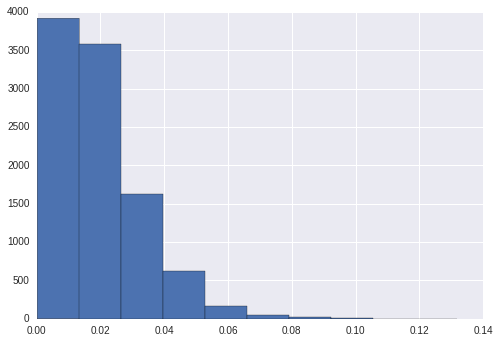In :
I_obs

Out:
array([[   1,    3,    0,    1,    0,    0,    0,    0,    1],
[   4,   13,    7,   18,    1,    2,    0,    1,    4],
[   3,   12,    2,   14,    0,    1,    1,    2,    5],
[   4,   10,    2,   17,    0,    2,    2,    2,    2],
[   6,   15,    7,   19,    1,    3,    1,    7,    6],
[  19,   27,   20,   34,    0,    7,    2,   13,    8],
[   9,   27,    6,   26,    1,    1,    1,    6,    8],
[  13,   27,   13,   20,    1,    4,    2,    5,    2],
[  28,   32,   16,   21,    2,    6,    1,    9,    9],
[  42,   39,   46,   31,    6,   17,    2,   13,   18],
[  93,   69,   72,   40,    4,   18,    6,   19,   26],
[ 157,   95,  153,   64,   12,   47,    5,   31,   42],
[ 359,  183,  315,  169,   26,   95,   18,   76,   68],
[ 807,  363,  622,  282,   65,  234,   34,  162,  136],
[1168,  660, 1035,  388,   87,  398,   63,  257,  166],
[1442,  913, 1193,  536,  137,  430,   48,  318,  292],
[1350, 1051, 1255,  643,  116,  476,   68,  366,  339],
[1314,  933, 1261,  525,  160,  474,   91,  448,  339],
[1218,  773, 1061,  444,  146,  458,   75,  424,  320],
[ 712,  485,  629,  292,   80,  262,   67,  267,  214],
[ 368,  295,  382,  187,   47,  163,   26,  122,   92],
[ 181,  162,  192,  130,   27,   97,   10,   43,   65],
[ 122,  151,   88,  102,   14,   43,   10,   27,   36],
[  72,   95,   63,   64,    6,   36,    2,   15,   18],
[  32,   46,   39,   52,    7,   15,    2,   20,   14],
[  20,   42,   30,   42,    2,    9,    2,    8,   17],
[   7,   23,    5,   15,    1,    4,    3,    3,    7],
[   1,    1,    2,    1,    0,    1,    0,    0,    0]])
In :
obs_date = '1997-06-15'
obs_index = sp_counts_2w.index <= obs_date
I_obs_t = I_obs[obs_index]

In :
np.sum(I_obs_t, (0)) / float(I_obs_t.sum())

Out:
array([ 0.21114206,  0.20557103,  0.19164345,  0.16991643,  0.01559889,
0.06016713,  0.01281337,  0.06016713,  0.0729805 ])
In :
I_obs_t

Out:
array([[  1,   3,   0,   1,   0,   0,   0,   0,   1],
[  4,  13,   7,  18,   1,   2,   0,   1,   4],
[  3,  12,   2,  14,   0,   1,   1,   2,   5],
[  4,  10,   2,  17,   0,   2,   2,   2,   2],
[  6,  15,   7,  19,   1,   3,   1,   7,   6],
[ 19,  27,  20,  34,   0,   7,   2,  13,   8],
[  9,  27,   6,  26,   1,   1,   1,   6,   8],
[ 13,  27,  13,  20,   1,   4,   2,   5,   2],
[ 28,  32,  16,  21,   2,   6,   1,   9,   9],
[ 42,  39,  46,  31,   6,  17,   2,  13,  18],
[ 93,  69,  72,  40,   4,  18,   6,  19,  26],
[157,  95, 153,  64,  12,  47,   5,  31,  42]])
In :
I_obs_t.sum((0,1))

Out:
1795
In :
I_obs_t.sum(1).cumsum()

Out:
array([   6,   56,   96,  137,  202,  332,  417,  504,  628,  842, 1189,
1795])
In :
from pymc import MCMC, Matplot, AdaptiveMetropolis
from pymc import (Uniform, DiscreteUniform, Beta, Binomial, Normal,
CompletedDirichlet,
Poisson, NegativeBinomial, negative_binomial_like, poisson_like,
Lognormal, Exponential, binomial_like,
TruncatedNormal, Binomial, Gamma, HalfCauchy, normal_like,
MvNormalCov, Bernoulli, Uninformative,
Multinomial, rmultinomial, rbinomial,
Dirichlet, multinomial_like)
from pymc import (Lambda, observed, invlogit, deterministic, potential, stochastic,)

def measles_model(obs_date, confirmation=True, spatial_weighting=False,
all_traces=False):

n_periods, n_age_groups = I_obs.shape

### Confirmation sub-model

if confirmation:

# Specify priors on age-specific means
age_classes = np.unique(age_index)

mu = Normal("mu", mu=0, tau=0.0001, value=*len(age_classes))
sig = HalfCauchy('sig', 0, 25, value=1)
var = sig**2
cor = Uniform('cor', -1, 1, value=0)

# Build variance-covariance matrix with first-order correlation
# among age classes
@deterministic
def Sigma(var=var, cor=cor):
I = np.eye(len(age_classes))*var
E = np.diag(np.ones(len(age_classes)-1), k=-1)*var*cor
return I + E + E.T

# Age-specific probabilities of confirmation as multivariate normal
# random variables
beta_age = MvNormalCov("beta_age", mu=mu, C=Sigma,
value=*len(age_classes))
p_age = Lambda('p_age', lambda t=beta_age: invlogit(t))

@deterministic(trace=False)
def p_confirm(beta=beta_age):
return invlogit(beta[age_index])

# Confirmation likelihood
lab_confirmed = Bernoulli('lab_confirmed', p=p_confirm, value=y,
observed=True)

'''
Truncate data at observation period
'''
obs_index = sp_counts_2w.index <= obs_date
I_obs_t = I_obs[obs_index]

# Index for observation date, used to index out values of interest
# from the model.
t_obs = obs_index.sum() - 1

if confirmation:

@stochastic(trace=all_traces, dtype=int)
def I(value=(I_obs_t*0.5).astype(int), n=I_obs_t, p=p_age):
# Binomial confirmation process
return np.sum([binomial_like(xi, ni, p) for xi,ni in zip(value,n)])

age_dist_init = np.sum(I.value, 0)/ float(I.value.sum())
else:

I = I_obs_t
age_dist_init = np.sum(I, 0) / float(I.sum())

assert I.shape == (t_obs +1, n_age_groups)

# Calcuate age distribution from observed distribution of infecteds to date
_age_dist = Dirichlet('_age_dist', np.ones(n_age_groups),
value=age_dist_init[:-1]/age_dist_init.sum())
age_dist = CompletedDirichlet('age_dist', _age_dist)
@potential
def age_dist_like(p=age_dist, I=I):
return multinomial_like(I.sum(0), I.sum(), p)
#     age_dist = age_dist_init

# Transmission parameter
beta = Uniform('beta', 1, 100, value=10)

# Downsample annual series to observed age groups
downsample = lambda x: np.array([x[s].mean() for s in age_slices])

# Weakly-informative prior on proportion susceptible being
# between 0 and 0.07
p_susceptible = Beta('p_susceptible', 2, 100)

# Estimated total initial susceptibles
S_0 = Binomial('S_0', n=int(N), p=p_susceptible)

S = Lambda('S', lambda I=I, S_0=S_0: S_0 - I.cumsum(axis=0))

# Check shape
assert S.value.shape == (t_obs+1., n_age_groups)

S_t = Lambda('S_t', lambda S=S: S[-1])

# Susceptibles at time t, by age
S_age = Multinomial('S_age', S_t, age_dist)

# Force of infection
@deterministic
def lam(beta=beta, I=I, S=S, N=N):
return beta * I.sum(axis=1) * S.sum(axis=1) / N

# Check shape
assert lam.value.shape == (t_obs+1,) # n_age_groups)

# FOI in observation period
lam_t = Lambda('lam_t', lambda lam=lam: lam[-1])

# Poisson likelihood for observed cases
@potential
def new_cases(I=I, lam=lam):
#         return negative_binomial_like(I[1:].sum(1), lam[:-1], I[:-1].sum(1))
return poisson_like(I.sum(axis=1), lam)

'''
Vaccination targets
'''

@deterministic
def vacc_5(S=S_age):
# Vaccination of 5 and under
p = [0.95] + *(n_age_groups - 1)
return rbinomial(S, p)

# Proportion of susceptibles vaccinated
pct_5 = Lambda('pct_5',
lambda V=vacc_5, S=S_age: V.sum()/S.sum())

@deterministic
def vacc_15(S=S_age):
# Vaccination of 15 and under
p = [0.95]*3 + *(n_age_groups - 3)
return rbinomial(S, p)

# Proportion of susceptibles vaccinated
pct_15 = Lambda('pct_15',
lambda V=vacc_15, S=S_age: V.sum()/S.sum())

@deterministic
def vacc_30(S=S_age):
# Vaccination of 30 and under
p = [0.95]*6 + *(n_age_groups - 6)
return rbinomial(S, p)

# Proportion of 30 and under susceptibles vaccinated
pct_30 = Lambda('pct_30',
lambda V=vacc_30, S=S_age: V.sum()/S.sum())

@deterministic
# Vaccination of adults under 30 (and young kids)
p = [0.95, 0, 0, 0, 0.95, 0.95] + *(n_age_groups - 6)
return rbinomial(S, p)

# Proportion of adults under 30 (and young kids)
lambda V=vacc_adult, S=S_age: V.sum()/S.sum())

return locals()


Run models for June 15 and July 15 observation points, both with and without clinical confirmation.

In :
db = 'ram'
n_iterations = 100000
n_burn = 50000


June 15, with lab confirmation

In :
model = measles_model

In :
model_june = MCMC(model('1997-06-15'), db=db, dbname='model_june')
#                                                 model_june.p_susceptible])

In :
model_june.sample(n_iterations, n_burn)

 [-----------------100%-----------------] 100000 of 100000 complete in 425.0 sec

July 15, with lab confirmation

In :
model_july = MCMC(model('1997-07-15'), db=db, dbname='model_july')
# model_july.use_step_method(AdaptiveMetropolis, [model_july.beta, model_july.p_susceptible])

In :
model_july.sample(n_iterations, n_burn)

 [-----------------100%-----------------] 100000 of 100000 complete in 428.3 sec

June 15, no lab confirmation

In :
model_june_noconf = MCMC(model('1997-06-15',
confirmation=False),
db=db, dbname='model_june_noconf')
# model_june_noconf.use_step_method(AdaptiveMetropolis, [model_june_noconf.β, model_june_noconf.p_susceptible])

In :
model_june_noconf.sample(n_iterations, n_burn)

 [-----------------100%-----------------] 100000 of 100000 complete in 76.3 sec

July 15, no lab confirmation

In :
model_july_noconf = MCMC(model('1997-07-15',
confirmation=False),
db=db, dbname='model_july_noconf')

In :
model_july_noconf.sample(n_iterations, n_burn)

 [-----------------100%-----------------] 100000 of 100000 complete in 81.0 sec

## Summary of model output¶

Distance weighting parameter for june model with confirmation

In :
Matplot.plot(model_june.p_susceptible)

Plotting p_susceptible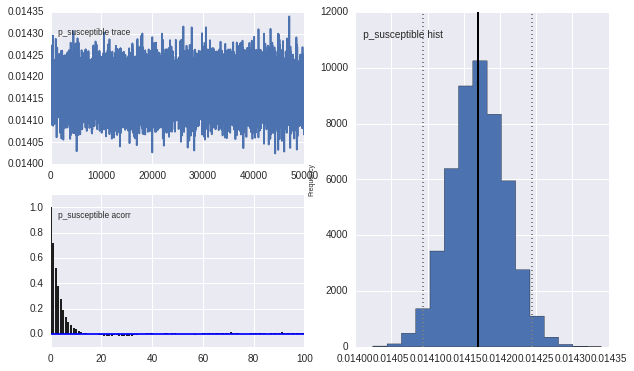In :
Matplot.plot(model_june.beta)

Plotting beta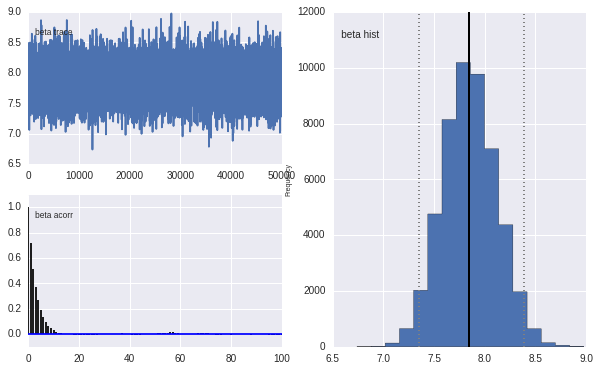Lab confirmation rates, June model

In :
p_age = pd.DataFrame(model_june.p_age.trace(), columns=age_groups)

f, axes = plt.subplots(figsize=(14,6))
sb.boxplot(data=p_age, linewidth=0.3, fliersize=0, ax=axes,
color=sb.color_palette("coolwarm", 5),
order=age_group.categories)
axes.set_ylabel('Confirmation rate')
axes.set_xlabel('Age group')

Out:
<matplotlib.text.Text at 0x7f64b1c63278>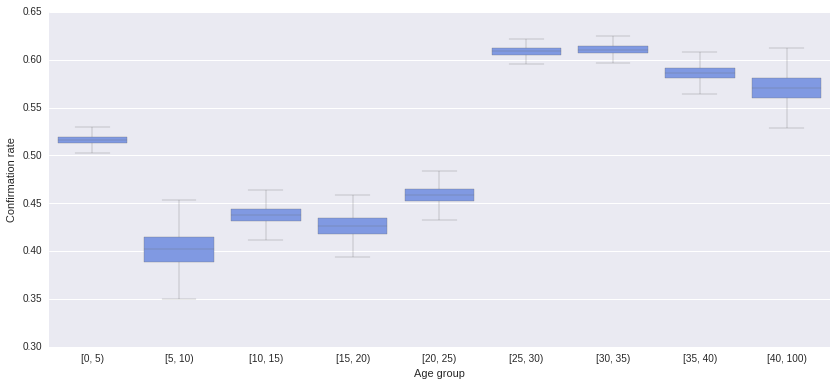Proportion of population susceptible, June model.

In :
Matplot.plot(model_july.beta)

Plotting beta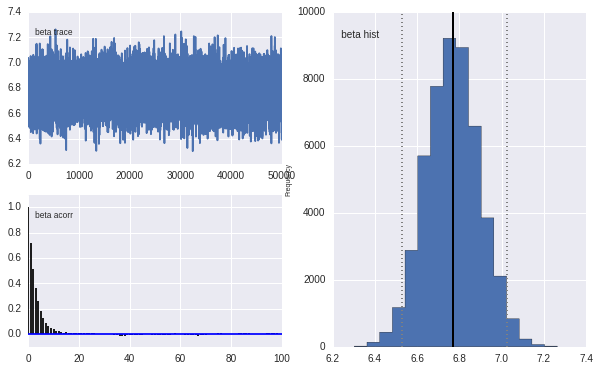Proportion of population susceptible, June model with no confirmation correction

In :
Matplot.plot(model_june_noconf.p_susceptible)

Plotting p_susceptible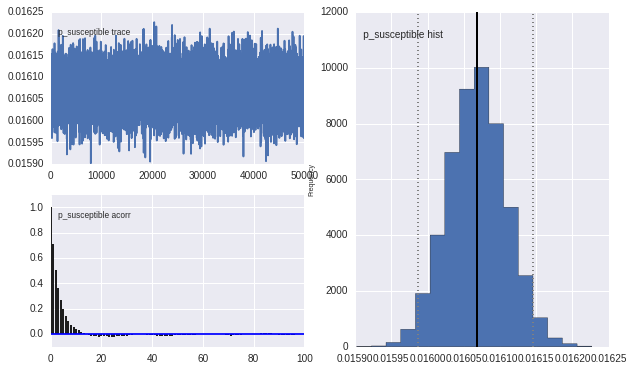Epidemic intensity estimates at June and July, per district.

In :
Matplot.plot(model_june.lam_t)

Plotting lam_t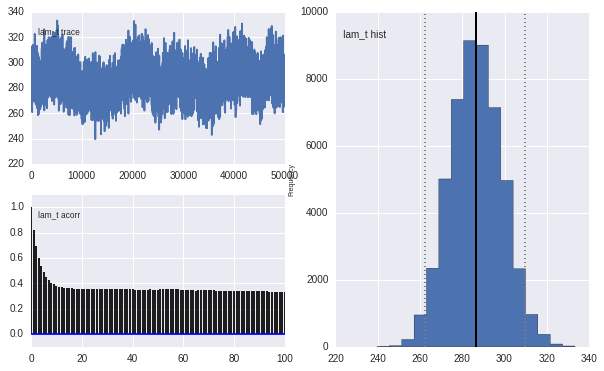In :
Matplot.plot(model_july.lam_t)

Plotting lam_t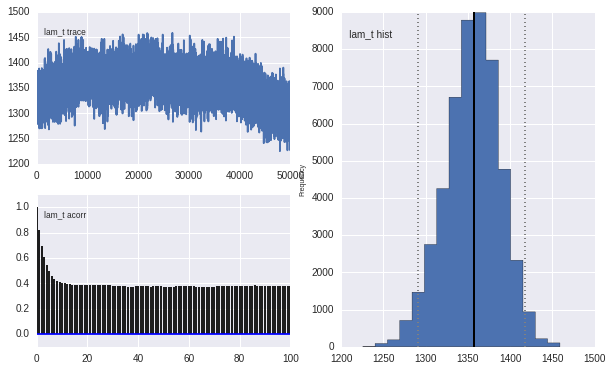Epidemic intensity for lab- versus clinical-confirmation models

In :
lam_june = model_june.lam.stats()

fig, axes = plt.subplots(2, 1, sharey=True)

axes.plot(lam_june['quantiles'].T, 'b-', alpha=0.4)
axes.set_ylabel('Epidemic intensity')
axes.set_xlabel('time (2-week periods)')
axes.set_title('Lab confirmation')

lam_june_noconf = model_june_noconf.lam.stats()

axes.plot(lam_june_noconf['quantiles'].T, 'b-', alpha=0.4)
axes.set_ylabel('Epidemic intensity')
axes.set_xlabel('time (2-week periods)')
axes.set_title('Clinical confirmation')

plt.tight_layout()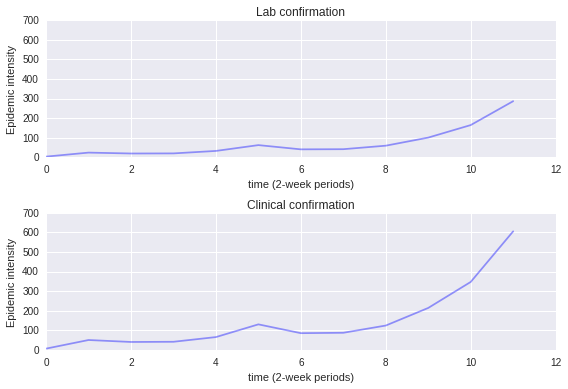In :
model_june.S_age.trace()

Out:
array([[27135, 25373, 23611, 25416,  2677, 11117,  2516,  8950, 10866]])
In :
S_age_june = pd.DataFrame(model_june.S_age.trace().squeeze(), columns=age_groups).unstack().reset_index()
S_age_june.columns = 'Age', 'Iteration', 'S'
S_age_june['Confirmation'] = 'Lab'

In :
S_age_june = pd.DataFrame(model_june.S_age.trace().squeeze(), columns=age_groups).unstack().reset_index()
S_age_june.columns = 'Age', 'Iteration', 'S'
S_age_june['Confirmation'] = 'Lab'

S_age_june_noconf = pd.DataFrame(model_june_noconf.S_age.trace().squeeze(), columns=age_groups).unstack().reset_index()
S_age_june_noconf.columns = 'Age', 'Iteration', 'S'
S_age_june_noconf['Confirmation'] = 'Clinical'

S_age_june = pd.concat([S_age_june, S_age_june_noconf], ignore_index=True)

In :
S_age_july = pd.DataFrame(model_july.S_age.trace().squeeze(), columns=age_groups).unstack().reset_index()
S_age_july.columns = 'Age', 'Iteration', 'S'
S_age_july['Confirmation'] = 'Lab'

S_age_july_noconf = pd.DataFrame(model_july_noconf.S_age.trace().squeeze(), columns=age_groups).unstack().reset_index()
S_age_july_noconf.columns = 'Age', 'Iteration', 'S'
S_age_july_noconf['Confirmation'] = 'Clinical'

S_age_july = pd.concat([S_age_july, S_age_july_noconf], ignore_index=True)


Numbers of suscepibles in each age group, under lab vs clinical confirmation

In :
g = sb.factorplot("Age", "S", "Confirmation", S_age_june, kind="box",
palette="hls", size=6, aspect=2, linewidth=0.3, fliersize=0,
order=age_group.categories)
g.despine(offset=10, trim=True)
g.set_axis_labels("Age Group", "Susceptibles");

/usr/local/lib/python3.4/dist-packages/matplotlib/__init__.py:892: UserWarning: axes.color_cycle is deprecated and replaced with axes.prop_cycle; please use the latter.
warnings.warn(self.msg_depr % (key, alt_key))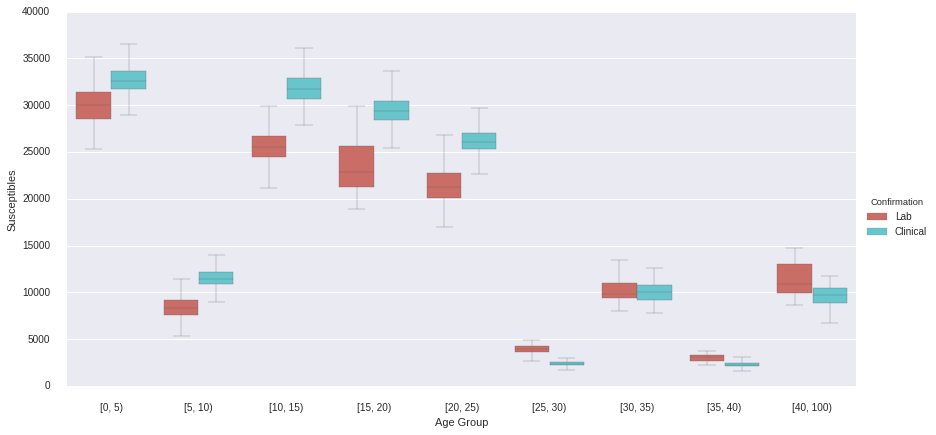In :
june_lam = pd.DataFrame(model_june.lam_t.trace()).unstack().reset_index()
june_lam.columns = ('district', 'iteration', 'λ')
june_lam['month'] = 'June'

In :
june_lam_noconf = pd.DataFrame(model_june_noconf.lam_t.trace()).unstack().reset_index()
june_lam_noconf.columns = ('district', 'iteration', 'λ')
june_lam_noconf['month'] = 'June'

In :
july_lam = pd.DataFrame(model_july.lam_t.trace()).unstack().reset_index()
july_lam.columns = ('district', 'iteration', 'λ')
july_lam['month'] = 'July'

In :
july_lam_noconf = pd.DataFrame(model_july_noconf.lam_t.trace()).unstack().reset_index()
july_lam_noconf.columns = ('district', 'iteration', 'λ')
july_lam_noconf['month'] = 'July'

In :
confirmed_lam = june_lam.append(july_lam, ignore_index=True)

In :
june_means = june_lam.groupby('district')['λ'].mean()
june_means.sort(ascending=False)

/usr/local/lib/python3.4/dist-packages/ipykernel/__main__.py:2: FutureWarning: sort is deprecated, use sort_values(inplace=True) for for INPLACE sorting
from ipykernel import kernelapp as app

In :
july_means = july_lam.groupby('district')['λ'].mean()
july_means.sort(ascending=False)

/usr/local/lib/python3.4/dist-packages/ipykernel/__main__.py:2: FutureWarning: sort is deprecated, use sort_values(inplace=True) for for INPLACE sorting
from ipykernel import kernelapp as app

In [ ]:
sorted_districts = june_means.index.values


Epidemic intensity by district in June and July (with lab confirmation), sorted by June means.

In [ ]:
sb.set_context("talk", font_scale=0.8)

f, (ax_1, ax_2) = plt.subplots(2, 1, figsize=(12,6), sharey=True, sharex=True)

sb.boxplot('district', 'λ', data=june_lam, ax=ax_1, linewidth=0.5,
fliersize=0, color='r', order=sorted_districts)
# ax_1.hlines(1, xmin=0, xmax=93, linestyles='dashed', linewidth=0.2)
ax_1.set_xticks([])
ax_1.set_xlabel('')
ax_1.set_ylabel('June')
ax_1.set_title(r'Epidemic intensity (λ) estimates, ordered by June means')

sb.boxplot('district', 'λ', data=july_lam, ax=ax_2, linewidth=0.5,
fliersize=0, color='r', order=sorted_districts)
# ax_2.hlines(1, xmin=0, xmax=93, linestyles='dashed', linewidth=0.2)
ax_2.set_xticks([])
ax_2.set_ylabel('July')

f.tight_layout()


Epidemic intensity by district in June for lab-confirmed and clinical-confirmed, sorted by lab-confirmed means.

In [ ]:
f, (ax_1, ax_2) = plt.subplots(2, 1, figsize=(12,6), sharey=True, sharex=True)

sb.boxplot('district', 'λ', data=june_lam, ax=ax_1, linewidth=0.5,
fliersize=0, color='r', order=june_means.index.values)
# ax_1.hlines(1, xmin=0, xmax=93, linestyles='dotted', linewidth=0.75)
ax_1.set_xticks([])
ax_1.set_xlabel('')
ax_1.set_ylabel('Lab')
ax_1.set_title(r'June epidemic intensity (λ) estimates, ordered by lab-confirmed means')

sb.boxplot('district', 'λ', data=june_lam_noconf, ax=ax_2, linewidth=0.5,
fliersize=0, color='r', order=june_means.index.values)
# ax_2.hlines(1, xmin=0, xmax=93, linestyles='dotted', linewidth=0.75)
ax_2.set_xticks([])
ax_2.set_ylabel('Clinical')

f.tight_layout()


Epidemic intensity by district in July for lab-confirmed and clinical-confirmed, sorted by lab-confirmed means.

In [ ]:
july_means = july_lam.groupby('district')['λ'].mean()
july_means.sort(ascending=False)

In [ ]:
f, (ax_1, ax_2) = plt.subplots(2, 1, figsize=(12,6), sharey=True, sharex=True)

sb.boxplot('district', 'λ', data=july_lam, ax=ax_1, linewidth=0.5,
fliersize=0, color='r', order=july_means.index.values)
# ax_1.hlines(1, xmin=0, xmax=93, linestyles='dotted', linewidth=0.75)
ax_1.set_xticks([])
ax_1.set_xlabel('')
ax_1.set_ylabel('Lab')
# ax_1.set_yticks(np.arange(13, step=2))
ax_1.set_title(r'July epidemic intensity (λ) estimates, ordered by lab-confirmed means')

sb.boxplot('district', 'λ', data=july_lam_noconf, ax=ax_2, linewidth=0.5,
fliersize=0, color='r', order=sorted_districts)
# ax_2.hlines(1, xmin=0, xmax=93, linestyles='dotted', linewidth=0.75)
ax_2.set_xticks([])
ax_2.set_ylabel('Clinical')

f.tight_layout()

In :
model_june.summary(['pct_5', 'pct_15', 'pct_30', 'pct_adult'])

pct_5:

Mean             SD               MC Error        95% HPD interval
------------------------------------------------------------------
0.207            0.013            0.001            [ 0.185  0.232]

Posterior quantiles:

2.5             25              50              75             97.5
|---------------|===============|===============|---------------|
0.184            0.197           0.207          0.217         0.231

pct_15:

Mean             SD               MC Error        95% HPD interval
------------------------------------------------------------------
0.546            0.02             0.002            [ 0.513  0.584]

Posterior quantiles:

2.5             25              50              75             97.5
|---------------|===============|===============|---------------|
0.509            0.53            0.544          0.563         0.581

pct_30:

Mean             SD               MC Error        95% HPD interval
------------------------------------------------------------------
0.793            0.014            0.001            [ 0.768  0.818]

Posterior quantiles:

2.5             25              50              75             97.5
|---------------|===============|===============|---------------|
0.764            0.782           0.793          0.804         0.816

Mean             SD               MC Error        95% HPD interval
------------------------------------------------------------------
0.305            0.012            0.001            [ 0.281  0.325]

Posterior quantiles:

2.5             25              50              75             97.5
|---------------|===============|===============|---------------|
0.282            0.295           0.306          0.314         0.326


In :
june_coverage = pd.DataFrame({name: model_june.trace(name)[:] for name in ['pct_5', 'pct_15', 'pct_30', 'pct_adult']})
june_coverage['Month'] = 'June'
june_coverage['Confirmation'] = 'Lab'

In :
june_noconf_coverage = pd.DataFrame({name: model_june_noconf.trace(name)[:] for name in ['pct_5', 'pct_15', 'pct_30', 'pct_adult']})
june_noconf_coverage['Month'] = 'June'
june_noconf_coverage['Confirmation'] = 'Clinical'

july_coverage = pd.DataFrame({name: model_july.trace(name)[:] for name in ['pct_5', 'pct_15', 'pct_30', 'pct_adult']})
july_coverage['Month'] = 'July'
july_coverage['Confirmation'] = 'Lab'

july_noconf_coverage = pd.DataFrame({name: model_july_noconf.trace(name)[:] for name in ['pct_5', 'pct_15', 'pct_30', 'pct_adult']})
july_noconf_coverage['Month'] = 'July'
july_noconf_coverage['Confirmation'] = 'Clinical'

In :
coverage = pd.concat([june_coverage, june_noconf_coverage, july_coverage, july_noconf_coverage],
ignore_index=True)

In :
sb.factorplot(row="Month", col="Confirmation", data=coverage, kind='box',
row_order=['June', 'July'],
order=['pct_5', 'pct_15', 'pct_30', 'pct_adult'],
palette="YlGnBu_d", linewidth=0.7, fliersize=0, aspect=1.25).despine(left=True)

/usr/local/lib/python3.4/dist-packages/matplotlib/__init__.py:892: UserWarning: axes.color_cycle is deprecated and replaced with axes.prop_cycle; please use the latter.
warnings.warn(self.msg_depr % (key, alt_key))

Out:
<seaborn.axisgrid.FacetGrid at 0x7f464480c2e8>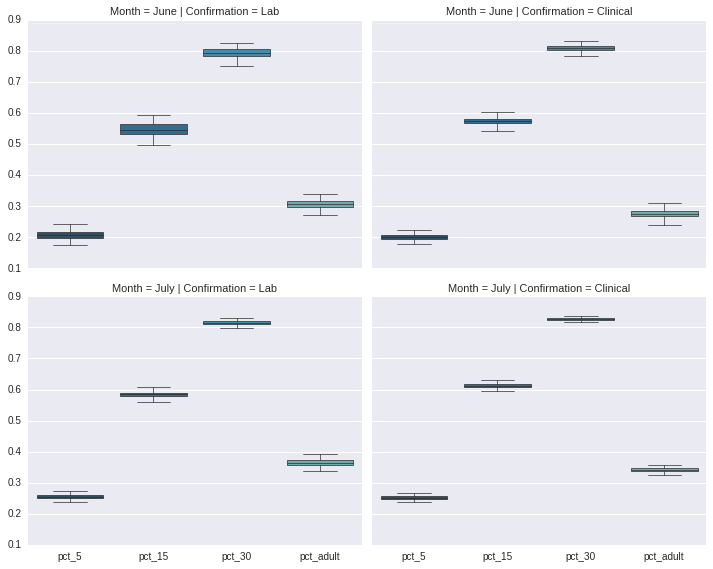In :
sb.factorplot(row="Month", col="Confirmation", data=coverage, kind='box',
row_order=['June', 'July'],
order=['pct_5', 'pct_15', 'pct_30', 'pct_adult'],
palette="YlGnBu_d", linewidth=0.7, fliersize=0, aspect=1.25).despine(left=True)

/usr/local/lib/python3.4/dist-packages/matplotlib/__init__.py:892: UserWarning: axes.color_cycle is deprecated and replaced with axes.prop_cycle; please use the latter.
warnings.warn(self.msg_depr % (key, alt_key))

Out:
<seaborn.axisgrid.FacetGrid at 0x7f64990997b8>In :
axes = sb.boxplot(data=june_coverage, order=['pct_5', 'pct_15', 'pct_30', 'pct_adult'],
color=sb.color_palette("coolwarm", 5))
axes.set_xticklabels(['Under 5', 'Under 15', 'Under 30', 'Under 5 + 20-30'])
axes.set_ylabel('% susceptibles vaccinated')
sb.despine(offset=10, trim=True)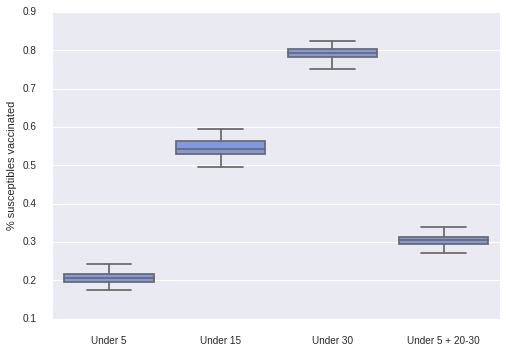In :
model_june_noconf.summary(['pct_5', 'pct_15', 'pct_30', 'pct_adult'])

pct_5:

Mean             SD               MC Error        95% HPD interval
------------------------------------------------------------------
0.199            0.01             0.001            [ 0.181  0.221]

Posterior quantiles:

2.5             25              50              75             97.5
|---------------|===============|===============|---------------|
0.179            0.194           0.199          0.205         0.22

pct_15:

Mean             SD               MC Error        95% HPD interval
------------------------------------------------------------------
0.573            0.01             0.001            [ 0.554  0.593]

Posterior quantiles:

2.5             25              50              75             97.5
|---------------|===============|===============|---------------|
0.553            0.565           0.573          0.581         0.592

pct_30:

Mean             SD               MC Error        95% HPD interval
------------------------------------------------------------------
0.807            0.009            0.001            [ 0.79   0.824]

Posterior quantiles:

2.5             25              50              75             97.5
|---------------|===============|===============|---------------|
0.789            0.801           0.807          0.813         0.823

Mean             SD               MC Error        95% HPD interval
------------------------------------------------------------------
0.275            0.013            0.001            [ 0.249  0.301]

Posterior quantiles:

2.5             25              50              75             97.5
|---------------|===============|===============|---------------|
0.247            0.266           0.274          0.284         0.3


In :
model_july.summary(['pct_5', 'pct_15', 'pct_30', 'pct_adult'])

pct_5:

Mean             SD               MC Error        95% HPD interval
------------------------------------------------------------------
0.255            0.007            0.001            [ 0.243  0.27 ]

Posterior quantiles:

2.5             25              50              75             97.5
|---------------|===============|===============|---------------|
0.24             0.251           0.255          0.26          0.269

pct_15:

Mean             SD               MC Error        95% HPD interval
------------------------------------------------------------------
0.584            0.009            0.001            [ 0.568  0.6  ]

Posterior quantiles:

2.5             25              50              75             97.5
|---------------|===============|===============|---------------|
0.567            0.578           0.584          0.59          0.599

pct_30:

Mean             SD               MC Error        95% HPD interval
------------------------------------------------------------------
0.815            0.006            0.001            [ 0.803  0.827]

Posterior quantiles:

2.5             25              50              75             97.5
|---------------|===============|===============|---------------|
0.803            0.811           0.816          0.819         0.827

Mean             SD               MC Error        95% HPD interval
------------------------------------------------------------------
0.365            0.01             0.001            [ 0.346  0.385]

Posterior quantiles:

2.5             25              50              75             97.5
|---------------|===============|===============|---------------|
0.343            0.358           0.365          0.372         0.384


In :
model_july_noconf.summary(['pct_5', 'pct_15', 'pct_30', 'pct_adult'])

pct_5:

Mean             SD               MC Error        95% HPD interval
------------------------------------------------------------------
0.252            0.006            0.001            [ 0.239  0.264]

Posterior quantiles:

2.5             25              50              75             97.5
|---------------|===============|===============|---------------|
0.239            0.248           0.252          0.256         0.264

pct_15:

Mean             SD               MC Error        95% HPD interval
------------------------------------------------------------------
0.613            0.007            0.001            [ 0.602  0.626]

Posterior quantiles:

2.5             25              50              75             97.5
|---------------|===============|===============|---------------|
0.602            0.608           0.612          0.617         0.627

pct_30:

Mean             SD               MC Error        95% HPD interval
------------------------------------------------------------------
0.827            0.004            0.0              [ 0.821  0.835]

Posterior quantiles:

2.5             25              50              75             97.5
|---------------|===============|===============|---------------|
0.821            0.825           0.827          0.83          0.835

Mean             SD               MC Error        95% HPD interval
------------------------------------------------------------------
0.342            0.006            0.001            [ 0.331  0.355]

Posterior quantiles:

2.5             25              50              75             97.5
|---------------|===============|===============|---------------|
0.33             0.338           0.342          0.346         0.354


In :
Matplot.summary_plot(model_june.p_age)

Could not calculate Gelman-Rubin statistics. Requires multiple chains of equal length.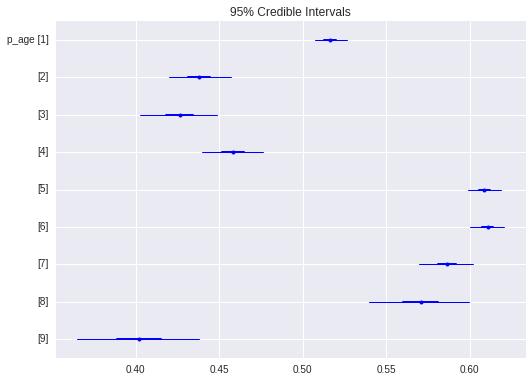## Mapping spatial effects¶

In [ ]:
from mpl_toolkits.basemap import Basemap
import geopandas as gpd

lllat=-24
urlat=-23.3
lllon=-47
urlon=-46.3

SP_base = Basemap(ax=None, lon_0=(urlon + lllon) / 2, lat_0=(urlat + lllat) / 2,
llcrnrlat=lllat, urcrnrlat=urlat, llcrnrlon=lllon, urcrnrlon=urlon,
resolution='i',
epsg='4326')

In [ ]:
SP_dist = gpd.GeoDataFrame.from_file('Sao Paulo/Brazil_full/BRA_adm3.shp').to_crs({'proj': 'longlat',
'ellps': 'WGS84',
'datum': 'WGS84'})

In [ ]:
SP_dist['DIST_NAME'] = [trans.trans(_).upper() for _ in SP_dist.NAME_3]

In [ ]:
λ_june = pd.Series(model_june.λ_t.stats()['mean'], index=sp_districts)

In [ ]:
λ_june

In [ ]:
SP_dist_merged = SP_dist.merge(pd.DataFrame(λ_june, columns=['λ']), left_on='DIST_NAME', right_index=True)

In [ ]:
measles_onset_conf = measles_data[CONFIRMED].groupby(['DISTRICT','ONSET']).size().unstack(level=0).fillna(0).sum()

In [ ]:
measles_onset_conf

In [ ]:
_rates = measles_onset_conf/sp_pop.sum(1)

In [ ]:
SP_dist_conf = SP_dist.merge(pd.DataFrame(_rates, columns=['rate']), left_on='DIST_NAME', right_index=True)


Estimated expected value for infecteds, by district

In [ ]:
from matplotlib.pyplot import cm

map_fig = plt.figure(figsize=(16,12))
map_ax = plt.gca()
SP_base.drawcoastlines()
SP_base.drawrivers()

SP_dist_merged.plot(column='λ', colormap=cm.Reds, axes=map_ax)


Observed confirmed cases, by district

In [ ]:
map_fig = plt.figure(figsize=(16,12))
map_ax = plt.gca()
SP_base.drawcoastlines()
SP_base.drawrivers()

SP_dist_conf.plot(column='rate', colormap=cm.Reds, axes=map_ax)